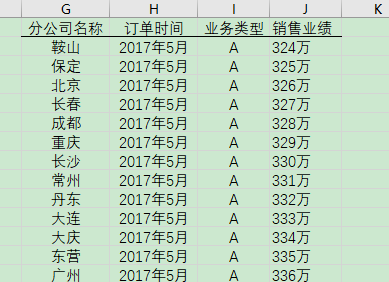1. 右键单击数据所在页的 sheet 标签。选择’查看代码’。（或者按快捷键 ALT + F11）
2. 复制下面的代码到新打开的窗口中。
3. 点击复制完代码的页面工具栏中的‘运行->运行子过程/用户窗体’。（或点击快捷键F5）
4. 执行后会弹出窗口提示“请输入拆分工作表的名称：”此时输入存储数据页面的工作簿名称（通常为“sheet1”）点击确认。
5. 弹出窗口提示“请输入拆分依据的列号（如A）：”此时按照我们的示例输入‘G’。
6. 弹出窗口提示“请输入拆分的开始行：”此时按照2（说明：行1为标题，从行2开始为数据。因此从第二行开始拆分）。
7. 点击确认开始拆分。
8. 弹出“拆分工作表完成”及结束。
9. 找到数据文件存储的路径。会发现一个名为“拆分出的表格”的文件夹。文件夹中已经保存好拆分完的表格了。

‘来自Geeker Xu加工整理’

Sub XXX_Click()

‘输入用户想要拆分的工作表

Dim sheet_name

sheet_name = Application.InputBox(“请输入拆分工作表的名称：”)

Worksheets(sheet_name).Select

‘输入获取拆分需要的条件列

Dim col_name

col_name = Application.InputBox(“请输入拆分依据的列号(如A):”)

‘输入拆分的开始行，要求输入的是数字

Dim start_row As Integer

start_row = Application.InputBox(prompt:=”请输入拆分的开始行:”, Type:=1)

‘暂停屏幕更新

Application.ScreenUpdating = False

‘工作表的总行数

Dim end_row

end_row = Worksheets(sheet_name).Range(“A65536”).End(xlUp).Row

‘遍历计算所有拆分表，每个拆分表的格式为”表名称,表行数”

‘对于二维数组，ReDim只能扩充最后一维，因此sheet_map行不变，扩充列

Dim sheet_map(), sheet_index

ReDim sheet_map(1, 0)

sheet_map(0, 0) = Range(col_name & start_row).Value

sheet_map(1, 0) = 1

sheet_index = 0

With Worksheets(sheet_name)

Dim row_count, temp, i

row_count = 0

For i = start_row + 1 To end_row

temp = Range(col_name & i).Value

If temp = Range(col_name & (i - 1)).Value Then

sheet_map(1, sheet_index) = sheet_map(1, sheet_index) + 1

Else

ReDim Preserve sheet_map(1, sheet_index + 1)

sheet_index = sheet_index + 1

sheet_map(0, sheet_index) = temp

sheet_map(1, sheet_index) = 1

End If

Next

End With

‘根据前面计算的拆分表，拆分成单个文件

Dim row_index

row_index = start_row

For i = 0 To sheet_index

‘创建最终数据文件夹

Dim dir_name

dir_name = ThisWorkbook.Path & “\拆分出的表格\”

If Dir(dir_name, vbDirectory) = “” Then

MkDir (dir_name)

End If

‘创建新工作簿

Dim workbook_path

workbook_path = ThisWorkbook.Path & “\拆分出的表格\” & sheet_map(0, i) & “.xlsx”

ActiveWorkbook.SaveAs workbook_path

ActiveSheet.Name = sheet_map(0, i)

‘激活当前工作簿，ThisWorkbook表示当前跑代码的工作簿

ThisWorkbook.Activate

‘拷贝条目数据(即最前面不需要拆分的数据行)

Dim row_range

row_range = 1 & “:” & (start_row - 1)

Worksheets(sheet_name).Rows(row_range).Copy

Workbooks(sheet_map(0, i) & “.xlsx”).Sheets(1).Range(“A1”).PasteSpecial

‘拷贝拆分表的专属数据

row_range = row_index & “:” & (row_index + sheet_map(1, i) - 1)

Worksheets(sheet_name).Rows(row_range).Copy

Workbooks(sheet_map(0, i) & “.xlsx”).Sheets(1).Range(“A” & start_row).PasteSpecial

row_index = row_index + sheet_map(1, i)

‘保存文件

Workbooks(sheet_map(0, i) & “.xlsx”).Close SaveChanges:=True

Next

‘进行屏幕更新

Application.ScreenUpdating = True

MsgBox “拆分工作表完成”

End Sub
希望 Geeker Xu 的分享可以对您的工作带来方便。